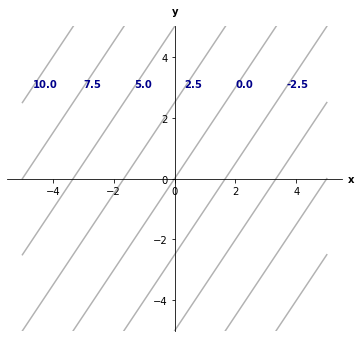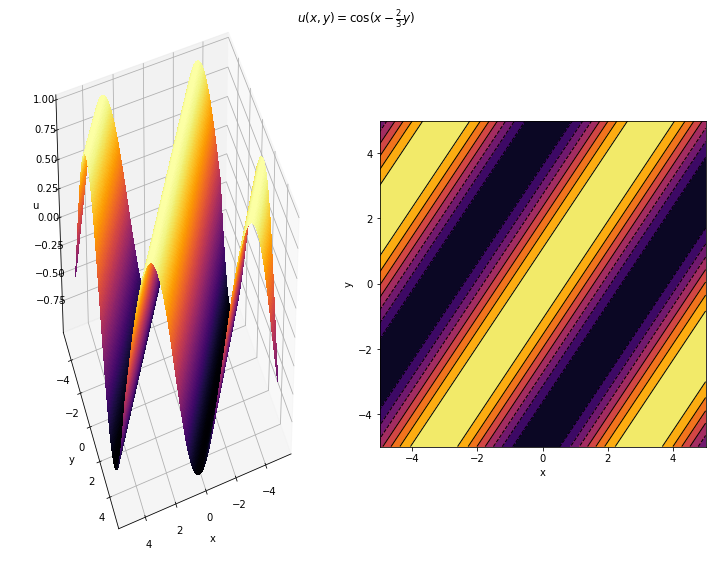# First-order linear PDEs#

Mathematics for Scientists and Engineers 2

In this section we will consider first-order linear PDEs for an unknown function $$u$$ of two variables:

$a(x,y)u_x + b(x,y)u_y = f(x, y, u)$

The method which we will use to find the solution of such PDEs is called the method of characteristics. This is a very useful method with an intuitive geometric interpretation and it can be applied to even more complicated PDEs, which we will not consider here.

## Method of characteristics#

The main idea behind this method is a popular one: we want to reduce the PDE to an ODE, which are in general much easier to solve. We do that by analysing the PDE along specially chosen curves in $$xy$$ plane, which we call characteristic curves or just characteristics. We parameterise them by the parameter $$s$$, such that $$x = x(s), y = y(s)$$ and we choose them such that the following system of ODEs is satisfied:

$\frac{dx}{ds} = a, \quad \frac{dy}{ds} = b, \quad \frac{du}{ds} = f$

Then after using the chain rule and substituting this, we have

$\frac{du}{ds} = \frac{\partial u}{\partial x} \frac{dx}{ds} + \frac{\partial u}{\partial y} \frac{dy}{ds} = f(x(s), y(s), u(s))$

This simplifies our problem quite a bit, since we have reduced the PDE to an ODE. Let us now look at a few examples.

### Example: constant coefficients, homogeneous#

Let us start with the simplest example, where $$a$$ and $$b$$ are constants and $$f \equiv 0$$:

$2u_x + 3u_y = 0, \qquad u(x, 0) = \cos x$

Setting

$\frac{dx}{ds} = 2, \quad \frac{dy}{ds} = 3$

and using the chain rule we get

$\frac{du}{ds} = 2u_x + 3u_y = 0$

This means that the PDE along the characteristic line $$(x(s), y(s))$$ is a constant. We can write the parametrization invariant form of the above equations as

$ds = \frac{dx}{2} = \frac{dy}{3}$

from which

$\frac{dx}{dy} = \frac{2}{3}$

So the characteristics are given by $$2y = 3x + c$$, where $$c$$ is an integration constant which will determine which characteristic line we are on. Let us plot a couple of them.

import numpy as np
import matplotlib.pyplot as plt

x = np.linspace(-5, 5, 51)
c = np.linspace(-10, 10, 9)

fig, ax = plt.subplots(1, 1, figsize=(6, 6))

for cc in c:
ax.plot(x, 3/2 * x + cc, 'k-', alpha=0.3)

if cc > -5:
ax.text(2 - cc/1.5, 3, cc, c='darkblue', weight='bold')

ax.text(-0.1, 5.4, 'y', weight='bold')
ax.text(5.7, -0.1, 'x', weight='bold')

ax.set_aspect('equal')
ax.spines['left'].set_position('zero')
ax.spines['bottom'].set_position('zero')
ax.spines['right'].set_visible(False)
ax.spines['top'].set_visible(False)
ax.set_ylim(-5, 5)

plt.show()Here we plotted characteristics for different values of $$c$$, which are written in bold along characteristics.

All characteristics are parallel to each other, of course, and since $$u$$ is constant along each characteristic line, $$u$$ will only vary if we jump from one characteristic to another. Since $$c$$ marks which characteristic we are sitting on, $$u$$ will therefore be a function of $$c$$.

$u(x(y), y) = f(c) = f(-3x + 2y)$

where $$f$$ is an arbitrary function of one variable.

Finally we need to use the auxiliary condition $$u(x, 0) = \cos x$$ to find the particular solution. For $$y=0$$:

$u(x, 0) = f(-3x) = \cos (x)$

Let $$w = -3x$$. Then:

\begin{align}\begin{aligned} f(w) = \cos (-w/3) .\\Hence the solution is\\ u(x, t) = f(-3x + 2y) = \cos(x - \frac{2}{3}y) \end{aligned}\end{align}
from mpl_toolkits.mplot3d import Axes3D

x = np.linspace(-5, 5, 201)
y = np.linspace(-5, 5, 201)
X, Y = np.meshgrid(x, y)

f = np.cos(X - 2/3 * Y)

fig = plt.figure(figsize=(10, 8))

ax1.plot_surface(X, Y, f, rstride=1, cstride=1, cmap='inferno', antialiased=False)
ax2.contourf(f, cmap='inferno', extent=[-5, 5, -5, 5])
ax2.contour(f, colors='k', extent=[-5, 5, -5, 5], linewidths=1.)

ax1.view_init(40, 70)
ax2.set_aspect('equal')

for ax in (ax1, ax2):
ax.set_xlabel('x')
ax.set_ylabel('y')
ax1.set_zlabel('u')

fig.suptitle(r'$u(x,y) = \cos (x - \frac{2}{3}y)$')

plt.tight_layout()
plt.show()Notice that each of the contours is a characteristic, since $$u$$ is constant along a characteristic.

Important

A first-order linear PDE of the form

$au_x + bu_y = 0$

has a general solution of the form

$u(x, y) = f(bx - ay)$

where $$f$$ is an arbitrary function of one variable.

## Example: general case#

Let us now consider an inhomogeneous linear problem with variable coefficients.

$(x + y)u_x + y u_y = xyu$

with the boundary condition $$u(x,y) = e^{x^2/4}$$ for $$y = x$$.